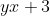# Problem Study (Analytic Geometry)

Find the equation of the line passing through the vertices of this curve.
Solution

Let the vertices be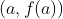and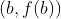where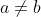.

and
The gradient of tangent to the curve is

At vertices, the tangents are parallel.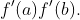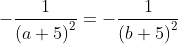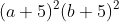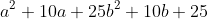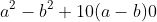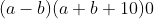Since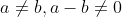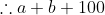and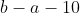.Since the line passing through vertices is perpendicular to the respective tangents, its gradient is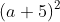.
Hence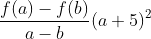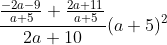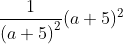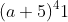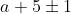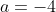(or)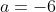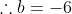(or)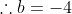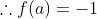(or)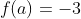and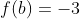(or)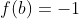Therefore, the vertices are (– 4, – 1) and (– 6, – 3).
Hence the equation of required line is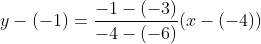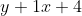(or)